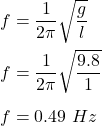## A pendulum has a 0.35\ \text{kg}0.35 kg0, point, 35, space, start text, k, g, end text mass oscillating at a small angle from a light string

Question

A pendulum has a 0.35\ \text{kg}0.35 kg0, point, 35, space, start text, k, g, end text mass oscillating at a small angle from a light string of length 1.0\ \text{m}1.0 m1, point, 0, space, start text, m, end text. What is the frequency of oscillation?

in progress 0
2 weeks 2021-08-29T18:50:28+00:00 1 Answers 0 views 0

The frequency of oscillation of the simple pendulum is 0.49 Hz.

Explanation:

Given that,

Mass of the simple pendulum, m = 0.35 kg

Length of the string to which it is attached, l = 1 m

We need to find the frequency of oscillation. The frequency of oscillation of the simple pendulum is given by :So, the frequency of oscillation of the simple pendulum is 0.49 Hz. Hence, this is the required solution.